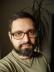Excel Column

Write a script that accepts a number and returns the Excel Column Name it represents and vice-versa.

Excel columns start at A and increase lexicographically using the 26 letters of the English alphabet, A..Z. After Z, the columns pick up an extra “digit”, going from AA, AB, etc., which could (in theory) continue to an arbitrary number of digits. In practice, Excel sheets are limited to 16,384 columns.

Example

Input Number: 28
Output: AB

Output: 30

This seemed like a simple base 10 to base 26 conversion and back. I started by installing Math::Base::Convert, Math::BaseConvert, Math::BaseCnv, and Convert::AnyBase to quickly discover they wouldn’t help me much. What Excel uses for column names is a weird 26 digit system that lacks a symbol for zero, but has a symbol for 26 (or for 1026). It’s called the bijective base-26 numeration system. The interesting fact about such systems is that digit addition, subtraction, and multiplication work the same way as in our common system (division is a bit problematic).

Converting a column name to its number is easy. Start from the left, add the number corresponding to the letter to the result, then multiply it by 26 and continue with the next letter.

Converting a number to the column name is a bit more complex because of the missing zero symbol. In each step, we decrement the remaining number before adding it modulo 26 to the result and dividing it by 26. For example, to get the name of the 789th column:

To convert    | Decremented | Modulo 26 | Result
--------------+-------------+-----------+-------
789 |         788 |         8 |    I
788 / 26 = 30 |          29 |         3 |   DI
29 / 26 =  1 |           0 |         0 |  ADI

What’s missing is to recognise which way we want to convert, but a simple regex can do that:

#!/usr/bin/perl
use warnings;
use strict;

sub convert {
my (\$in) = @_;
my \$r;
if (\$in =~ /^[0-9]+\$/) {
\$r = "";
while (\$in) {
substr \$r, 0, 0, chr(--\$in % 26 + ord 'A');
\$in = int(\$in / 26);
}

} elsif (\$in =~ /^[A-Z]+\$/) {
\$r = 0;
while (\$in) {
\$r *= 26;
\$r += ord(substr \$in, 0, 1, "") + 1 - ord 'A';
}

} else {
die "Invalid input: \$in!\n";
}

return \$r
}

use Test::More;

is convert(1), 'A', 'A';
is convert(26), 'Z', 'Z';
is convert(27), 'AA', 'AA';
is convert(52), 'AZ', 'AZ';
is convert(53), 'BA', 'BA';

is convert('A'), 1, 'A';
is convert('Z'), 26, 'Z';
is convert('AA'), 27, 'AA';
is convert('AZ'), 52, 'AZ';
is convert('BA'), 53, 'BA';

is convert(28), 'AB', 'encode';

done_testing();

Another way how to verify the correctness was to run Excel (well, in my case, LibreOffice Calc) (warning: YouTube, but without sound).

Find Numbers

Write a script that accepts list of positive numbers (@L) and two positive numbers \$X and \$Y.

The script should print all possible numbers made by concatenating the numbers from @L, whose length is exactly \$X but value is less than \$Y.

Example

Input:

@L = (0, 1, 2, 5);
\$X = 2;
\$Y = 21;
Output:

10, 11, 12, 15, 20

The first thing I noticed was 0 was considered positive. If we only used positive numbers, i.e. excluded zero, the solution would be a bit simpler, because zero has a special property when considering the length of a number: concatenation of zero and a number has the same length as the original number (i.e. concat(0, 12) = 12).

I decided to use a recursive function that extended the list of numbers by concatenations of all of their possible pairs. The trick was to keep the numbers that were still shorter than the target length separated from the numbers that had already reached the target length. It prevented the program from blindly trying to combine the shortest numbers again and again ad infinitum.

I used a hash for the concatenated numbers to remove duplicates. Numbers that were too long were simply thrown away. Once no new concatenated number was generated (i.e. the number of shorter numbers hadn’t changed), the recursion ended and the result was filtered by the remaining condition.

#!/usr/bin/perl
use warnings;
use strict;

sub extend {
my (\$length, \$short, \$long) = @_;
my %next;
undef @next{@\$short};
for my \$i (0 .. \$#\$short) {
for my \$j (0 .. \$#\$short) {
my \$new = 0 + (\$short->[\$i] . \$short->[\$j]);
next if \$length < length \$new;
if (length(\$new) < \$length) {
undef \$next{\$new};
} else {
undef \$long->{\$new};
}
}
}
return keys %\$long if @\$short == keys %next;

return extend(\$length, [keys %next], \$long)
}

sub find {
my (\$length, \$greater, @list) = @_;
my @long = grep \$length == length \$_, @list;
my %long; undef @long{@long} if @long;
return grep \$greater > \$_,
extend(\$length, \@list, \%long);
}

use Test::More;
use Test::Deep;

cmp_deeply [ find(2, 21, 0, 1, 2, 5) ],
bag(10, 11, 12, 15, 20);

cmp_deeply [ find(4, 3111, 1, 2, 3) ],
bag(glob '{{1,2}{1,2,3}}{{1,2,3}{1,2,3}}');

cmp_deeply [ find(5, 20000, 0, 1) ],
bag(glob '1{0,1}{0,1}{0,1}{0,1}');

cmp_deeply [ find(3, 222, 2) ],
[];

cmp_deeply [ find(10, 2000000022, 0, 2) ],
bag(2000000000, 2000000002, 2000000020);

cmp_deeply [ find(5, 30000, 1, 20, 300) ],
bag(11111, 11120, 11201, 11300, 12011, 12020,
13001, 20111, 20120, 20201, 20300);

cmp_deeply [ find(3, 789, 123, 456) ],
bag(123, 456);

done_testing();I blog about Perl.